# NCERT Solutions For Class 10 Maths Chapter 7 Exercise 7.2

Go back to  'Coordinate Geometry'

## Chapter 7 Ex.7.2 Question 1

Find the coordinates of the point which divides the join of $$(-1, 7)$$ and $$(4, -3)$$ in the ratio $$2:3$$.

### Solution

Reasoning:

The coordinates of the point $$P(x, y)$$ which divides the line segment joining the points $$A(x_1, y_1)$$ and $$B(x_2, y_2)$$, internally, in the ratio $$m_1 : m_2$$ is given by the Section Formula

\begin{align}\!P(x,y) \!=\! \left[ {\frac{{mx_2} \!+\! {nx_1}}{{m \!+ n}},\frac{{my_2} \!+\! {ny_1}}{{m} +\! {n}}}\! \right]\! \end{align}

What is Known?

The $$x$$ and $$y$$ co-ordinates of the points which is to be divided in the ratio $$2:3$$

What is Unknown?

The coordinates of the point which divides the join of $$(-1, 7)$$ and $$(4, -3)$$ in the ratio $$2:3$$

Steps:

Given,

• Let $$P(x, y)$$ be the required point.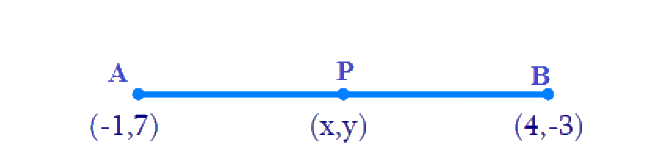• Let $$A(-1, 7)$$ and $$B(4, -3)$$
• $$m: n = 2:3$$
• Hence
• $$x_1 = -1$$
• $$y_1 = 7$$
• $$x_2 = 4$$
• $$y_2 = -3$$

By Section formula

\begin{align}\!P(x,y) \!=\! \left[ {\frac{{mx_2} \!+\! {nx_1}}{{m \!+ n}},\frac{{my_2} \!+\! {ny_1}}{{m} +\! {n}}}\! \right]\!\;\;\dots(1) \end{align}

By substituting the values in the Equation (1)

\begin{align}x &= \frac{{2 \times 4 + 3x( - 1)}}{{2 + 3}} \\x &= \frac{{8 - 3}}{5}\\x &= \frac{5}{5} = 1\\\\ y &= \frac{{2 \times ( - 3) + 3 \times 7}}{{2 + 3}} \\y &= \frac{{ - 6 + 21}}{5} \\y &= \frac{{15}}{5} = 3\end{align}

Therefore, the co-ordinates of point $$P$$ are $$(1, 3)$$.

## Chapter 7 Ex.7.2 Question 2

Find the coordinates of the points of trisection of the line segment joining $$(4, -1)$$ and $$(-2, -3)$$.

### Solution

Reasoning:

The coordinates of the point $$P(x, y)$$ which divides the line segment joining the points $$A(x1, y1)$$ and $$B(x2, y2)$$, internally, in the ratio $$m1 : m2$$ is given by the Section Formula.

What is Known?

The $$x$$ and $$y$$ co-ordinates of the line segment joining $$(4, -1)$$ and $$(-2, -3)$$.

What is Unknown?

The coordinates of the points of trisection of the line segment joining $$(4, -1)$$ and $$(-2, -3)$$.

Steps: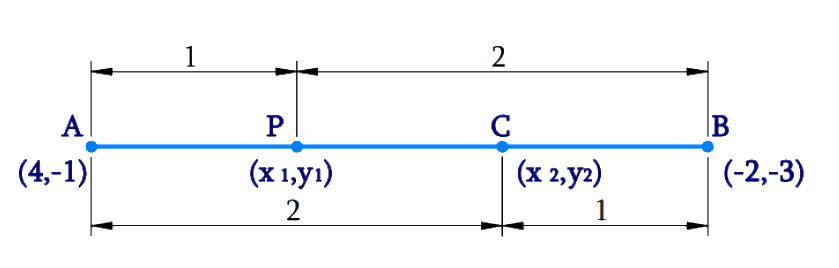Given,

• Let line segment joining the points be $$A(4, -1)$$ and $$B(-2, -3)$$.
• Let $$P (x_1, y_1)$$ and $$Q(x_2, y_2)$$ be the points of trisection of the line segment joining the given points i.e., $$AP = PQ = QB$$

By Section formula

\begin{align}P(x,y)\! =\! \left[\! {\frac{mx_2 + nx_1}{m+n},\!{\frac{my_2 +\! ny_1}{m+ n}}}\right]\;\;\dots(1) \end{align}

Therefore, by observation point $$P$$ divides $$AB$$ internally in the ratio $$1:2$$.

• Hence $$m: n = 1:2$$

By substituting the values in the Equation (1)

\begin{align}x_1 & \! = \! \frac{{1 \! \times \! ( - 2) \! + \! 2 \! \times \! 4}}{{1 \! + \! 2}}\\x_1 & \! = \! \frac{{ - 2 \! + \! 8}}{3} \\& \! = \! \frac{6}{3} \\& \! = \! 2\\\\ y_1 & \! = \! \frac{{1 \! \times \! ( - 3) \! + \! 2 \! \times \! ( - 1)}}{{1 \! + \! 2}}\\ y_1 & \! = \! \frac{{ - 3 - 2}}{3} \\& \! = \! \frac{{ - 5}}{3}\end{align}

Therefore,

\begin{align}{\text{P}}\left( x_1,y_1 \right) = \left( {2,\frac{{ - 5}}{3}} \right)\end{align}

Therefore, by observation point $$Q$$ divides $$AB$$ internally in the ratio $$2:1$$.

• Hence $$m:n = 2:1$$

By substituting the values in the Equation (1)

\begin{align}x_2 & \! = \! \frac{{2 \! \times \! ( - 2) \! + \! 1 \! \times \! 4}}{{2 \! + \! 1}}\\x_2 & \! = \! \frac{{ - 4 \! + \! 4}}{3} \\& \! = \! 0\\\\y_2 & \! = \! \frac{{2x( - 3) \! + \! 1 \! \times \! ( - 1)}}{{2 \! + \! 1}}\\ y_2 & \! = \! \frac{{ - 6 - 1}}{3} \\& \! = \! \frac{{ - 7}}{3}\end{align}

Therefore,

\begin{align}Q( x_2,y_2) = \left( {0, - \frac{7}{3}} \right)\end{align}

Hence the points of trisection are

\begin{align}P( x_1,y_1) &= \left( {2,\frac{{ - 5}}{3}} \right) \\&\text{and}\;\\ Q(x_2,y_2) &= \left( {0, - \frac{7}{3}} \right)\end{align}

## Chapter 7 Ex.7.2 Question 3

To conduct Sports Day activities, in your rectangular shaped school ground $$ABCD$$, lines have been drawn with chalk powder at a distance of $$1\rm\,m$$ each. $$100$$ flower pots have been placed at a distance of $$1\rm\,m$$ from each other along $$AD$$, as shown in the following figure. Niharika runs \begin{align}\frac{1}{4}\end{align}th the distance $$AD$$ on the $$2^\rm {nd}$$ line and posts a green flag. Preet runs \begin{align}\frac{1}{5}\end{align}th the distance $$AD$$ on the eighth line and posts a red flag. What is the distance between both the flags? If Rashmi has to post a blue flag exactly halfway between the line segments joining the two flags, where should she post her flag?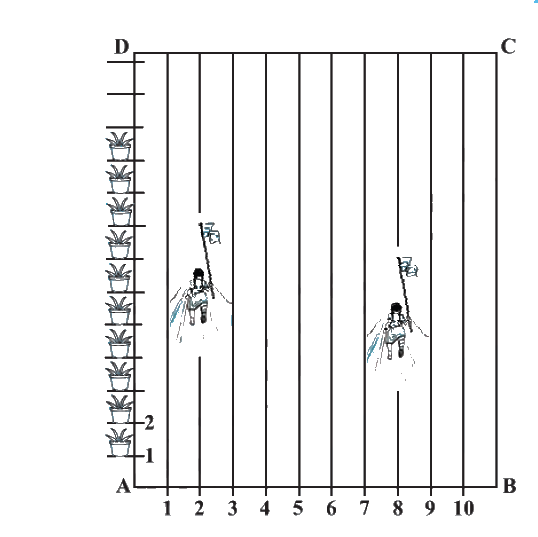### Solution

Reasoning:

The coordinates of the point $$P(x, y)$$ which divides the line segment joining the points $$A(x_1, y_1)$$ and $$B(x_2, y_2)$$, internally, in the ratio $$m_1 : m_2$$ is given by the Section Formula.

What is Known?

• The school ground $$ABCD$$ is rectangular shaped.
• Lines are drawn at a distance of $$1\rm\,m$$ each and $$100$$ flower pots have been placed at a distance of $$1\rm\,m$$ each along $$AD$$.
• The distance covered by Niharika and Preet on line $$AD$$.

What is Unknown?

• The distance between the flags posted by Niharika and Preet.
• The position on the line segment where Rashmi has to post the flag.

Steps:

From the Figure,

Given,

• By observation, that Niharika posted the green flag at of the distance $$P$$ i.e., \begin{align}\left( {\frac{1}{4} \times 100} \right)m = 25\,m\end{align} from the starting point of $$2^\rm{nd}$$ Therefore, the coordinates of this point $$P$$ is $$(2, 25)$$.
• Similarly, Preet posted red flag at $$\frac{{1}}{5}$$ of the distance $$Q$$ i.e., \begin{align}\left( {\frac{1}{5} \times 100} \right)m = 20\,m\end{align} from the starting point of $$8^\rm {th}$$ Therefore, the coordinates of this point $$Q$$ are $$(8, 20)$$

We know that the distance between the two points is given by the Distance Formula,

\begin{align}&\sqrt {{{\left( x_1 - x_2 \right)^2 + \left(y_1 - y_2 \right)}^2}} \;\;\dots(1)\end{align}

To find the distance between these flags $$PQ$$ by substituting the values in Equation (1),

\begin{align}PQ &= \sqrt {{{(8 - 2)}^2} + {{(25 - 20)}^2}} \\ &= \sqrt {36 + 25} \\ &= \sqrt {61}\text{ m} \end{align}

• The point at which Rashmi should post her blue flag is the mid-point of the line joining these points.
• Let this point be $$M \;(x, y)$$.

By Section formula

\begin{align} P(x,y)& \!=\! \left[ {\frac{{mx_2 \! + \! nx_1}}{{m \! + \! n}}\!,\!\frac{{my_2 \! + \! ny_1}}{{m \! + \! n}}} \!\right]\;\;\dots(2) \; \end{align}

\begin{align}x &= \frac{{2 + 8}}{2}, \qquad y = \frac{{25 + 20}}{2}\\x &= \frac{{10}}{2}, \qquad\quad\; y = \frac{{45}}{2}\\x &= 5, \qquad\qquad \;y = 22.5\end{align}

Therefore, Rashmi should post her blue flag at $$22.5\;\rm m$$ on $$5^\rm {th}$$ line

## Chapter 7 Ex.7.2 Question 4

Find the ratio in which the line segment joining the points $$(-3, 10)$$ and $$(6, -8)$$ is divided by $$(-1, 6)$$.

### Solution

Reasoning:

The coordinates of the point $$P(x, y)$$ which divides the line segment joining the points $$A(x_1, y_1)$$ and $$B(x_2, y_2)$$, internally, in the ratio $$m_1 : m_2$$ is given by the Section Formula.

\begin{align} P(x,y)& \!=\! \left[ {\frac{{mx_2 \! + \! nx_1}}{{m \! + \! n}}\!,\!\frac{{my_2 \! + \! ny_1}}{{m \! + \! n}}} \!\right]\end{align}

What is Known?

The $$x$$ and $$y$$ co-ordinates of the line segment which is divided by the point $$(-1, 6)$$.

What is Unknown?

The ratio in which the line segment joining the points $$(-3, 10)$$ and $$(6, -8)$$ is divided by $$(-1, 6).$$

Steps:

From the figure,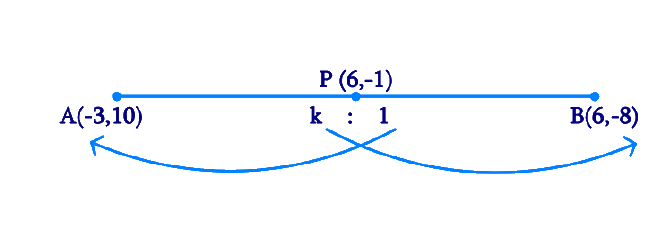Given,

• Let the ratio in which the line segment joining $$A(-3, 10)$$ and $$B(6, -8)$$ is divided by point $$P(-1, 6)$$ be $$k:1$$.

By Section formula

\begin{align} P(x,y)& \!=\! \left[ {\frac{{mx_2 \! + \! nx_1}}{{m \! + \! n}}\!,\!\frac{{my_2 \! + \! ny_1}}{{m \! + \! n}}} \!\right]\;\;\dots(2) \; \end{align}

Therefore,

\begin{align} - 1 &= \frac{{6k - 3}}{{k + 1}}\\ - k - 1 &= 6k - 3\\7k &= 2\end{align}

By Cross Multiplying & Transposing

\begin{align}k &= \frac{2}{7}\end{align}

Hence the point $$P$$ divides $$AB$$ in the ratio $$2:7$$

## Chapter 7 Ex.7.2 Question 5

Find the ratio in which the line segment joining $$A(1, -5)$$ and $$B(-4, 5)$$ is divided by the $$x$$-axis. Also find the coordinates of the point of division.

### Solution

Reasoning:

The coordinates of the point $$P(x, y)$$ which divides the line segment joining the points $$A(x_1, y_1)$$ and $$B(x_2, y_2)$$, internally, in the ratio $$m_1 : m_2$$ is given by the Section Formula.

What is Known?

The $$x$$ and $$y$$ co-ordinates of the line segment which is divided by the $$x$$-axis.

What is Unknown?

The ratio in which the line segment joining $$A(1, -5)$$ and $$B(-4, 5)$$ is divided by the $$x$$-axis and the coordinates of the point of division

Steps:

From the Figure,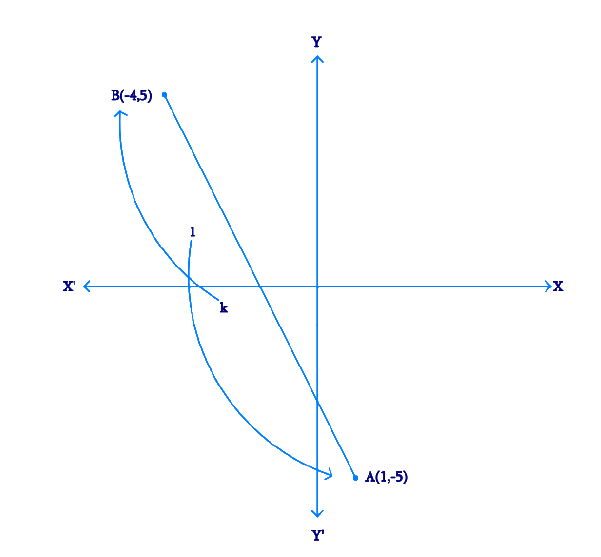Given,

• Let the ratio be $$k : 1$$.
• Let the line segment joining $$A(1, -5)$$ and $$B(-4, 5)$$

By Section formula

\begin{align}{{P(x,}}\,{{y)}} & \!=\! \left[\! {\frac{{{{m}}{{{x}}_2} \!+\! {{n}}{{{x}}_1}}}{{{{m}} \!+\! {{n}}}},\frac{{{{m}}{{{y}}_2} \!+\! {{n}}{{{y}}_1}}}{{{{m}} \!+\! {{n}}}}}\! \right] \;\;\dots(1) \\ &\begin{bmatrix}\text{By substituting the values} \\ \text{in Equation (1)}\end{bmatrix} \end{align}

Therefore, the coordinates of the point of division is \begin{align}\left[{\frac{{ - 4k + 1}}{{k + 1}},\;\frac{{5k - 5}}{{k + 1}}} \right]\end{align}

We know that $$y$$-coordinate of any point on $$x$$-axis is $$0$$.

\begin{align}∴\; \frac{{5{{k}} - 5}}{{{{k}} + 1}} &= 0\\\;\;\;5{{k}} - 5 &= 0\\\;\;\;\, \to 5{{k}}& = 5 \\&\!\!\!\!\!\!\!\!\!\!\!\!\!\!\!\!\!\!\! \begin{bmatrix}\text{By Cross Multiplying}\\\text { & Transposing}\end{bmatrix} \\\\{{k}} &= 1\end{align}

Therefore, $$x$$-axis divides it in the ratio $$1:1$$.

Division point

\begin{align}&= \left[ {\frac{{ - 4(1) + 1}}{{1 + 1}},\frac{{5(1) + 5}}{{1 + 1}}} \right]\\ &= \left[ {\frac{{ - 4 + 1}}{2},\frac{{5 + 5}}{2}} \right]\\ &= \left[ {\frac{{ - 3}}{2},0} \right]\end{align}

## Chapter 7 Ex.7.2 Question 6

If $$(1, 2)$$, $$(4, y)$$, $$(x, 6)$$ and $$(3, 5)$$ are the vertices of a parallelogram taken in order, find $$x$$ and $$y$$.

### Solution

Reasoning:

The coordinates of the point $$P(x, y)$$ which divides the line segment joining the points $$A(x_1, y_1)$$ and $$B(x_2, y_2)$$, internally, in the ratio $$m_1 : m_2$$ is given by the Section Formula.

\begin{align}{{P(x,}}\,{{y)}} & \!=\! \left[\! {\frac{{{{m}}{{{x}}_2} \!+\! {{n}}{{{x}}_1}}}{{{{m}} \!+\! {{n}}}},\frac{{{{m}}{{{y}}_2} \!+\! {{n}}{{{y}}_1}}}{{{{m}} \!+\! {{n}}}}}\! \right]\end{align}

What is the known?

The $$x$$ and $$y$$ co-ordinates of the vertices of the parallelogram.

What is the unknown?

The missing $$x$$ and $$y$$ co-ordinate.

Steps:

From the Figure,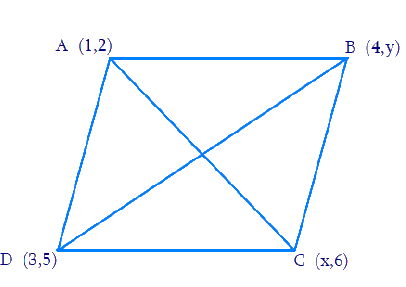Given,

• Let $$A (1, 2)$$, $$B (4, y)$$, $$C(x, 6)$$, and $$D(3, 5)$$ are the vertices of a parallelogram $$ABCD$$.
• Since the diagonals of a parallelogram bisect each other, Intersection point $$O$$ of diagonal $$AC$$ and $$BD$$ also divides these diagonals

Therefore, $$O$$ is the mid-point of $$AC$$ and $$BD$$.

If $$O$$ is the mid-point of $$AC$$, then the coordinates of $$O$$ are

\begin{align}\left( {\frac{{1 + x}}{2},\;\frac{{2 + 6}}{2}} \right) \Rightarrow \left( {\frac{{x + 1}}{2},\;4} \right)\end{align}

If $$O$$ is the mid-point of $$BD$$, then the coordinates of $$O$$ are

\begin{align}\left( {\frac{{4 + 3}}{2},\;\frac{{5 + y}}{2}} \right) \Rightarrow \left( {\frac{7}{2},\;\frac{{5 + y}}{2}} \right)\end{align}

Since both the coordinates are of the same point $$O$$,

\begin{align} &\therefore \frac{{x + 1}}{2} = \frac{7}{2}\;\;{\text{ and }}\;\;4 = \frac{{5 + y}}{2} \end{align}

\begin{align} &\Rightarrow x + 1 = 7\;\;{\text{ and }}\;\;5 + y = 8 \, \end{align} (By cross multiplying & transposing)

\begin{align} &\Rightarrow x = 6\;\;{\text{ and }}\;\; y = 3\end{align}

## Chapter 7 Ex.7.2 Question 7

Find the coordinates of a point $$A$$, where $$AB$$ is the diameter of circle whose center is $$(2, -3)$$ and $$B$$ is $$(1, 4)$$

### Solution

Reasoning:

The coordinates of the point $$P(x, y)$$ which divides the line segment joining the points $$A(x_1, y_1)$$ and $$B(x_2, y_2)$$, internally, in the ratio $$m_1 : m_2$$ is given by the Section Formula.

What is the known?

The $$x$$ and $$y$$ co-ordinates of the center of the circle and one end of the diameter $$B$$.

What is the unknown?

The coordinates of a point $$A$$.

Steps:

From the Figure,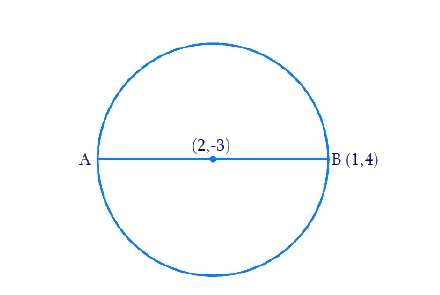Given,

• Let the coordinates of point $$A$$ be $$(x, y)$$.
• Mid-point of $$AB$$ is $$C$$ $$(2, -3)$$, which is the center of the circle.

\begin{align} \therefore \; (2, - 3) &= \left( {\frac{{x + 1}}{2},\frac{{y + 4}}{2}} \right) \end{align}

\begin{align} \Rightarrow \frac{{x + 1}}{2}& = 2\;\;{\text{ and }}\;\;\frac{{y + 4}}{2} = - 3 & & \end{align} (By Cross multiplying & transposing)

\begin{align} \Rightarrow x + 1 &= 4\;\;{\text{ and }}\;\;y + 4 = - 6 \end{align}

\begin{align} \Rightarrow x &= 3\;\;{\text{ and }}\;\;y = - 10\end{align}

Therefore, the coordinates of $$A$$ are $$(3, -10)$$

## Chapter 7 Ex.7.2 Question 8

If $$A$$ and $$B$$ are $$(-2, -2)$$ and $$(2, -4)$$, respectively, find the coordinates of $$P$$ such that \begin{align}AP= \frac{3}{7}AB\end{align} and $$P$$ lies on the line segment $$AB$$.

### Solution

Reasoning:

The coordinates of the point $$P(x, y)$$ which divides the line segment joining the points $$A(x_1, y_1)$$ and $$B(x_2, y_2)$$, internally, in the ratio $$m_1 : m_2$$ is given by the Section Formula.

\begin{align}{{P}}({{x}},{{y}}) \!=\! \left[\! {\frac{{{{m}}{{{x}}_2} \!+\! {{n}}{{{x}}_1}}}{{{{m}} \!+ \!{{n}}}}\!,\!\frac{{{{m}}{{{y}}_2} \!+\! {{n}}{{{y}}_1}}}{{{{m}} \!+\! {{n}}}}} \!\right] \! \end{align}

What is the known?

The $$x$$ and $$y$$ co-ordinates of the points $$A$$ and $$B$$.

The ratio in which $$P$$ divides $$AB$$.

What is the unknown?

Co-ordinates of $$P$$

Steps:

From the Figure,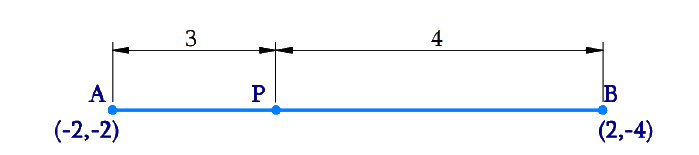Given,

• The coordinates of point $$A$$ and $$B$$ are $$(-2, -2)$$ and $$(2, -4)$$ respectively.
• \begin{align}{{AP}} = \frac{3}{7}{{AB}}\end{align}

Hence \begin{align}\frac{{{{AB}}}}{{{{AP}}}} = \frac{7}{3}\end{align}

We know that \begin{align}AB = AP + PB\end{align}

from figure,

\begin{align}\frac{{{{AP}} + {{PB}}}}{{{{AP}}}} &= \frac{{3 + 4}}{3}\\1 + \frac{{{{PB}}}}{{{{AP}}}}& = 1 + \frac{4}{3}\\\frac{{{{PB}}}}{{{{AP}}}}& = \frac{4}{3}\end{align}

Therefore, $$AP:PB = 3:4$$

Point $$P(x, y)$$ divides the line segment $$AB$$ in the ratio $$3:4.$$ Using Section Formula,

Coordinates of

\begin{align}P({{x}},{{y}}) & \! = \! \begin{bmatrix}\left({\frac{{3 \times 2 + 4 \times ( - 2)}}{{3 + 4}}}\right), \\ \left(\frac{{3 \times ( - 4) + 4 \times ( - 2)}}{{3 + 4}}\right) \end{bmatrix}\\ & \! = \! \left[ {\frac{{6 - 8}}{7},\;\frac{{ - 12 - 8}}{7}} \right]\\ & \! = \! \left[ { - \frac{2}{7},\; - \frac{{20}}{7}} \right]\end{align}

## Chapter 7 Ex.7.2 Question 9

Find the coordinates of the points which divide the line segment joining $$A (-2, 2)$$ and $$B(2, 8)$$ into four equal parts.

### Solution

Reasoning:

The coordinates of the point $$P(x, y)$$ which divides the line segment joining the points $$A(x_1, y_1)$$ and $$B(x_2, y_2)$$, internally, in the ratio $$m_1 : m_2$$ is given by the Section Formula.

What is the known?

The $$x$$ and $$y$$ co-ordinates of the points $$A$$ and $$B$$.

What is the unknown?

The coordinates of the points which divide the line segment joining $$A \;(-2, 2)$$ and $$B\;(2, 8)$$ into four equal parts.

Steps: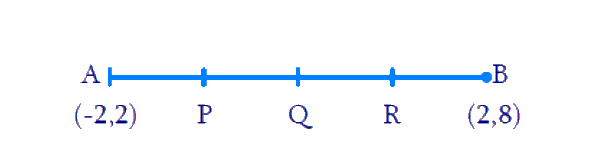From the Figure,

By observation, that points $$P$$, $$Q$$, $$R$$ divides the line segment $$A(-2, 2)$$ and $$B(2, 8)$$ into four equal parts

Point $$P$$ divides the line segment $$AQ$$ into two equal parts

Hence, Coordinates of

\begin{align}\!\! P \!&\!=\!\! \!\left[ \!{\frac{{\!1\! \times \!2 \!+\! 3 \!\times \!( - 2)}}{{1 + 3}}\!,\!\frac{{1 \times \!8\! + \!3\! \times\!2}}{{1 + 3}}}\!\! \right]\!\! \\&= \left[ { - 1,\;\frac{7}{2}} \right]\end{align}

Point $$Q$$ divides the line segment $$AB$$ into two equal parts

Coordinates of

\begin{align} Q &= \left[ {\frac{{2 + ( - 2)}}{2},\;\frac{{2 + 8}}{2}} \right]\\ &= (0,\;5)\end{align}

Point $$R$$ divides the line segment $$BQ$$ into two equal parts

Coordinates of

\begin{align}\!\! R &\!=\!\left[\! {\frac{{3\! \times\! 2\! + \!1 \!\times\! ( -\! 2)}}{{3 + 1}}\!,\!\frac{{3\! \times\! 8\! + \!1\! \times\! 2}}{{3 + 1}}}\! \right]\;\\ &=\left[1, \frac{13}{2}\right]\end{align}

## Chapter 7 Ex.7.2 Question 10

Find the area of a rhombus if its vertices are $$(3, 0)$$, $$(4, 5)$$, $$(-1, 4)$$ and $$(-2, -1)$$ taken in order.

[Hint: Area of a rhombus $$=$$ (product of its diagonals)]

### Solution

Reasoning:

A rhombus has all sides of equal length and opposite sides are parallel.

What is the known?

The $$x$$ and $$y$$ co-ordinates of the vertices of the rhombus.

What is the unknown?

The area of the rhombus

Steps: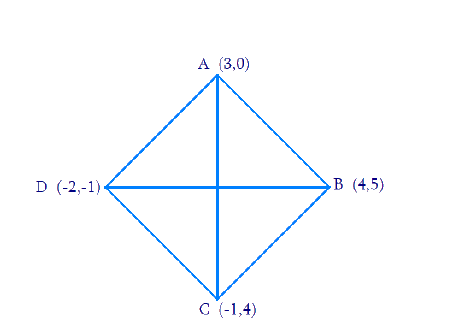From the Figure,

Given,

• Let $$A(3, 0)$$, $$B(4, 5)$$, $$C(-1, 4)$$ and $$D(-2, -1)$$ are the vertices of a rhombus $$ABCD$$.

We know that the distance between the two points is given by the Distance Formula,

\begin{align}&\sqrt {{{\left( {{{{x}}_1} - {{{x}}_2}} \right)}^2} + {{\left( {{{{y}}_1} - {{y}_2}} \right)}^2}}\;\;\dots(1)\end{align}

Therefore, distance between $$A(3, 0)$$ and $$C (-1, 4)$$ is given by

Length of diagonal

\begin{align} AC &= \sqrt {{{[3 - ( - 1)]}^2} + {{(0 - 4)}^2}} \\ &= \sqrt {16 + 16} = 4\sqrt 2 \end{align}

Therefore, distance between $$B(4, 5)$$ and $$D(-2, -1)$$ is given by

Length of diagonal

\begin{align} BD &= \sqrt {{{[4 - ( - 2)]}^2} + \left( {5 - {{( - 1)}^2}} \right.} \\& = \sqrt {36 + 36} \\&= 6\sqrt 2 \end{align}

\begin{align} &\text{Area of the rhombus} ABCD\\ &=\! \frac{1}{2}\!\! \times\!\! \text{(Product of lengths of diagonals)} \\&= \frac{1}{2} \times {\text{AC}} \times {\text{BD}}\end{align}

Therefore, area of rhombus

\begin{align}ABCD &= \frac{1}{2} \times 4\sqrt 2 \times 6\sqrt 2 \\ &= 24 \;\rm square\; units\end{align}